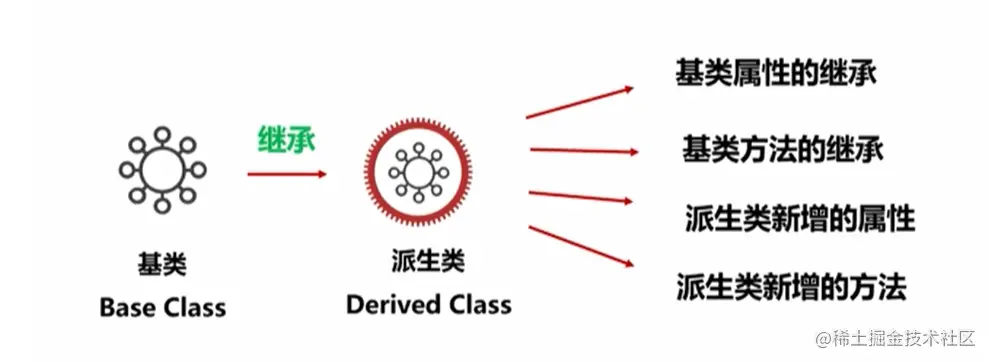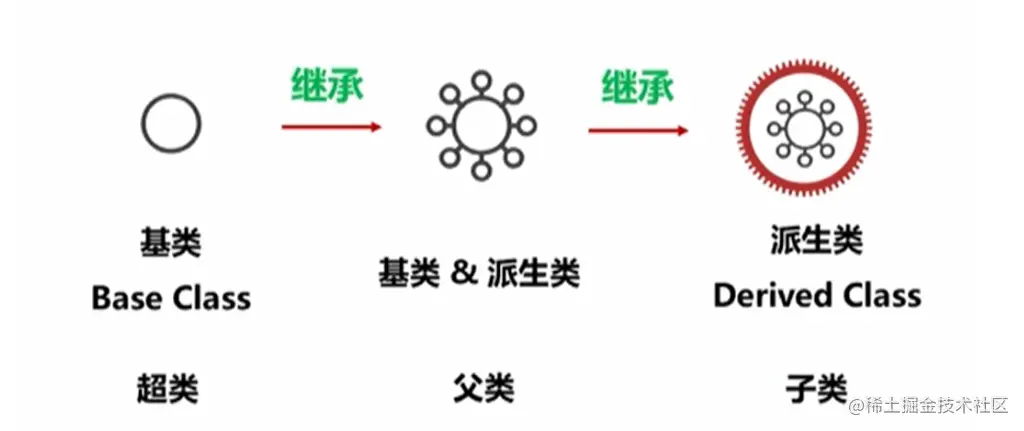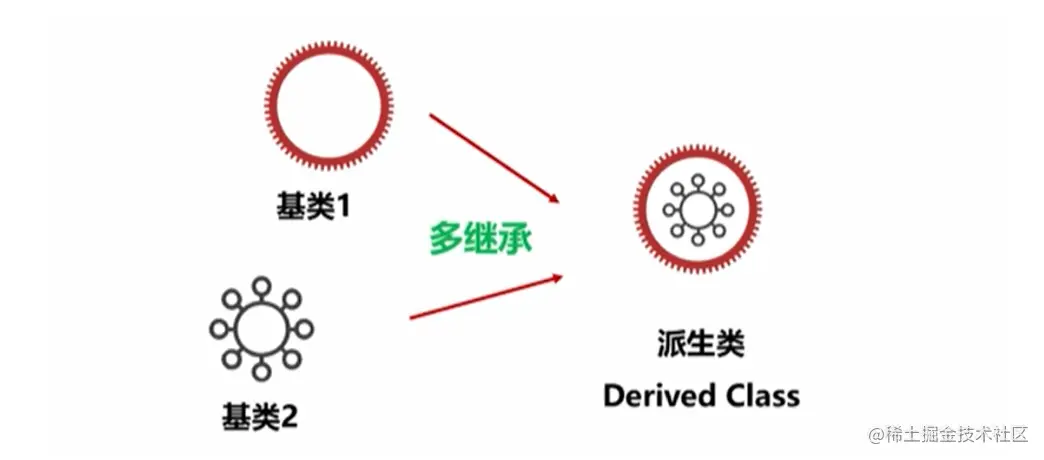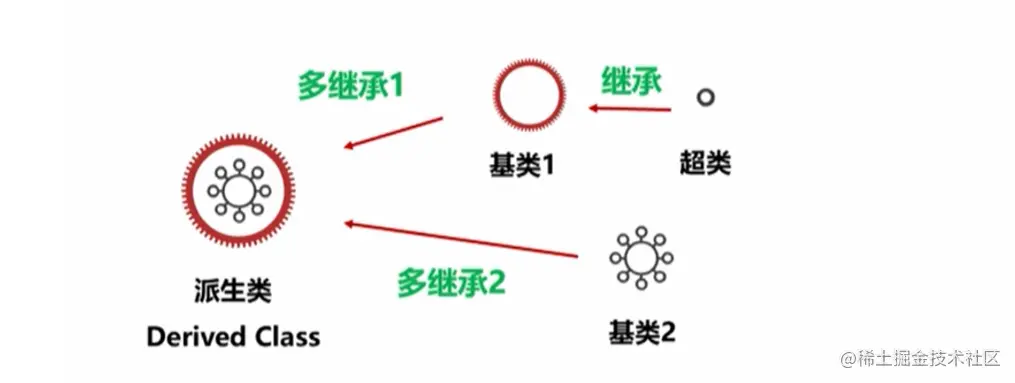# Python notes (12): inheritance such as object-oriented programming

2022-01-30 12:38:22

Hello everyone , I am a A bowl week , One doesn't want to be drunk ( Internal volume ) The front end of the . If you are lucky enough to get your favor , I'm very lucky ~

## Understanding of inheritance

Inherit （Inheritance ： High level abstraction of code reuse

• Inheritance is one of the essence of object-oriented design
• It realizes the high-level abstract code reuse based on classes
• Inheritance is a process in which a newly defined class can almost completely use the properties and methods of the original classWhether it's a base class or a derived class , It's just an inheritance statement , These are all ordinary Python class

You can also press subclasses 、 Parent class and superclass Division .

The most basic class is the base class , After one inheritance, the derived class , You can also inherit again , Another derived class ; Now the most basic class and the derived class inherited for the first time are the relationship between parent and child classes , Derived class the last derived class is also the relationship between parent class and child class , The most basic class and the last derived class belong to the relationship between superclass and subclassA derived class can not only inherit a base class , You can also inherit multiple base classes , This is the concept of multi inheritance## Construction of class inheritance

When a class inherits, the inheritance relationship is declared at the time of definition , The syntax is as follows

``````class < Derived class name >(< Base class name >):  #  The base class name can take the path :ModuleNama.BaseClassName
def __init__(self, < parameter list >):
< Sentence block >
...
Copy code ``````

Derived classes can directly use the properties and methods of the base class

• The properties of the base class are basically equal to those defined in the derived class
• Derived classes can directly use the class properties of the base class 、 Instance attributes
• Derived classes can directly use various methods of the base class
• When using class methods and class properties of a base class , To call... With the class name of the base class

The sample code

``````class TestClass:
def __init__(self, number):
self.sum_number = 0
for i in range(number + 1):
self.sum_number += i

def sum_num(self):
return self.sum_number

class HumanNameClass(TestClass):
def double_sum(self):
return self.sum_number * 2  #  Use of base class properties

value1 = HumanNameClass(100)
print(value1.sum_num())  # 5050  #  Use of base class instance methods
print(value1.double_sum())  # 10100  #  Use of derived class instance methods
Copy code ``````

Python There are two functions related to the judgment of inheritance relationship

function describe
`isinstance(object, classinfo)` Judge the object object Whether it is classinfo Instance or subclass instance of , return True perhaps False
`issubclass(class, classinfo)` Judgment parameters class Whether it is a type parameter classinfo Subclasses of , return True perhaps False.

``````print(isinstance(value1, TestClass))  # True
print(isinstance(value1, HumanNameClass))  # True
print(isinstance(value1, int))  # False
print(issubclass(HumanNameClass, TestClass))  # True
print(issubclass(TestClass, HumanNameClass))  # False
Copy code ``````

## Python The most basic class in

because Python Everything is an object , Any class is also an object 、Python All data types are also objects ;Python The most basic class of all classes provided by the language is object.

• object yes Python The most basic noun , There is no need to translate
• All definitions inherit by default object
• Reserved attributes and reserved methods are essentially object Class properties and methods

Sample code

``````print(object.__name__)  #  Print object Name   # object
print(object.__bases__)  #  Print object Inherited class name   # ()
print(object.__doc__)  #  Print object Class description   # The most base type
print(object.__module__)  #  Print object Name of the module   # builtins
print(object.__class__)  # object Corresponding class information   # <class 'type'>
Copy code ``````

Python The three elements of the object

• identification identity： Once the object is built, it will not change , use `id()` get , Usually memory address
• type type： Type of object , use `type()` get
• value value： Divided into variable mutable And immutable immutable Two kinds of

Two related to basic classes Python The built-in function

function / keyword describe
id(x) return x The logo of .CPython in id() Function to get the memory address of an object .
x `is` y Judge x and y Whether the identifications of are equal , return True or False, No judgment value

Overloading is the definition of a derived class to a base class property or method

• Property Override ： The derived class defines and uses an attribute with the same name as the base class
• Method overloading ： The derived class defines and uses a method with the same name as the base class

### Property Override

1. Give priority to the redefined properties and methods of derived classes
2. Then find the properties and methods of the base class
3. Looking for super class properties and methods

The sample code

``````class TestClass:
text = " This is the class attribute of the base class "

def __init__(self, number):
self.sum_number = 0
for i in range(number + 1):
self.sum_number += i

def sum_num(self):
return self.sum_number

class HumanNameClass(TestClass):
text = " This is the class attribute of the derived class "  #  Class attribute overload

def __init__(self, number):
self.sum_number = 1000  #  Instance property overload

def double_sum(self):
return self.sum_number * 2

value1 = HumanNameClass(100)
print(TestClass.text)  #  This is the class attribute of the base class
print(value1.text)  #  This is the class attribute of the derived class
print(value1.sum_num())  # 1000
Copy code ``````

Method overloading is the definition of a derived class to a base class method ; It is divided into full overload and incremental overload

Full reload ： Derived classes completely redefine methods with the same name as the base class

Directly define the method with the same name in the derived class

Sample code

``````class TestClass:
def __init__(self, number):
self.sum_number = 0
for i in range(number + 1):
self.sum_number += i

def sum_num(self):
return self.sum_number

class HumanNameClass(TestClass):

def sum_num(self):  #  Reconstruction of methods
return self.sum_number * 2

value1 = HumanNameClass(100)
print(value1.sum_num())  # 10100
Copy code ``````

Incremental reload ： Derived class extensions define methods with the same name as the base class , Grammatical structure

``````class < Derived class name >(< Base class name >):
def < Method name >(self, < parameter list >):
super().< Base class method name >(< parameter list >)
...
Copy code ``````

Incremental overloading uses `super()` function

Sample code

``````class TestClass:
def test_text(self):
print(" This is the method of the base class ")

class TestClass1(TestClass):
super().test_text()
print(" This is the statement in the new method ")

doc1 = TestClass()
doc2 = TestClass1()
print(doc1.test_text())
print(doc2.test_text())
'''
--- Output results ---
This is the method of the base class
None   #  Because the function does not return a value
This is the method of the base class
This is the statement in the new method
None  #  Because the function does not return a value

'''
Copy code ``````

## Multiple inheritance of classes

The construction of multiple inheritance is to declare the inheritance relationship at the time of definition , Grammatical structure

``````class < Class name >(< Base class name 1>, < Base class name 2>,..., < Base class name N>):  #  The base class name can take the path :ModuleNama.BaseClassName
def __init__(self, < parameter list >):
< Sentence block >
...
Copy code ``````

Python Depth first is adopted in multi inheritance , From left to right . The so-called depth first, from left to right, is to start from the leftmost , Find his base class , If the base class is not looking up , Until the most basic object Class has not been found yet , Just started looking to the right .All properties and methods are used according to “ Depth priority from left to right ” Method selection

Constructors also refer to the above principles ,`super()` Also refer to the above principles

The order of multiple base classes is the key

Sample code

``````class Test1:
def test(self):
text = " This is the base class 1"
return text

class Test2:
def test(self):
text = " This is the base class 2"
return text

class Test3(Test1, Test2):
pass

class Test4(Test2, Test1):
pass

value1 = Test3()
value2 = Test4()
print(value1.test())  #  This is the base class 1
print(value2.test())  #  This is the base class 2
Copy code ``````

Determine the output in sequence

Understanding of the concept of inheritance , Construction of class inheritance , I understand object yes Python The most basic class in

Overloading of class methods ： Reloading is similar to reloading class attributes ; Incremental overloading uses `super()` function

The method of multi inheritance is depth first , From left to right